# Test: Functions- 2

## 20 Questions MCQ Test Quantitative Reasoning for GMAT | Test: Functions- 2

Description
Attempt Test: Functions- 2 | 20 questions in 40 minutes | Mock test for Quant preparation | Free important questions MCQ to study Quantitative Reasoning for GMAT for Quant Exam | Download free PDF with solutions
QUESTION: 1

### If [x] denotes the greatest integer less than or equal to x, what is the value of [x]? (1) When x2 is divided by 9, the quotient is zero. (2) 2x2+9x+10=0

Solution:

Steps 1 & 2: Understand Question and Draw Inferences

Given:

• If x is an integer, then [x] = x
• If x is a decimal of the form a.bc… where a, b, c etc. are the units, tenths, hundredths . . . digits respectively of x, then [x] = a
• Example, if x = 2.45, then the greatest integer that is less than x is 2
• If x is a decimal of the form –a.bc . . . , where here a, b, c etc. are the units, tenths, hundredths . . . digits respectively of x, then [x] = -a – 1
• Example, if x = -2.45, then the greatest integer that is less than x is -3 (that is, -2 – 1)

To find: [x] = ?

• To answer this question, we need to know the value of x

Step 3: Analyze Statement 1 independently

(1) When x2 is divided by 9, the quotient is zero

• x2=9∗0+r , where remainder r is less than 9
• x2=r, where r is less than 9
• This means, x2 < 9
• That is, x2 - 32 < 0
• (x + 3)(x - 3) < 0
• From wavy line method, we know that the above inequality will be satisfied for:

-3 < x <3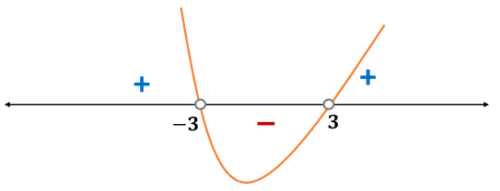If x lies between -3 and -2, then [x] = -3

If x lies between -2 and -1, then [x] = -2 and so on.

Thus, Statement 1 is not sufficient to find a unique value of [x]

Step 4: Analyze Statement 2 independently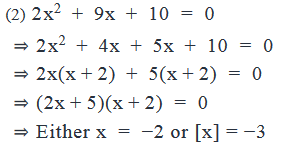Step 5: Analyze Both Statements Together (if needed)

From Statement 1: -3 < x < 3

From Statement 2: x = -2 or x = -2.5

Corresponding values of [x] = -2 or -3

Both values of x from Statement 2 conform to the inequality from Statement 1

So, even after combining the two statements, we still get two values of x, and correspondingly, two values of [x]

Thus, the two statements together are not sufficient to find a unique answer to the question.

QUESTION: 2

### For the positive integers k, m and n, k(m)n means that the remainder when m is divided by n is k. If k(13766)9 and p(137)k, where p is a positive integer, then p is equal to.

Solution:

Given:

• For positive integers k, m and n, k(m)n implies k is the remainder when m is divided by n
• k(13766)9 → k is the remainder when 13766 is divided by 9
• p(137)k → p is the remainder when 137 is divided by k
• p is a positive integer

To find: p = ?

Approach:

1. To find the value of p, we need to evaluate the expression p(137)k
• However, this expression also contains k
• So, we’ll be able to find the value of p only when we first know the value of k
2. We can find the value of k by evaluating the expression k(13766)9

Working Out:

• Finding the value of k
• k(13766)9 means that the remainder when 13766 is divided by 9 is k.
• Sum of digits of 13766 = 1+3+7+6+6 = 23
• So, remainder when 13766 is divided with 9 = Remainder that 23 leaves with 9 = 5
• Thus, k = 5
• Finding the value of p
• p(137)k means that the remainder when 137 is divided by k is p
• We’ve inferred above that k = 5
• So, the above expression means that when 137 is divided by 5, the remainder is p
• Now, 137 leaves a remainder of 2 with 5
• So, p= 2

Looking at the answer choices, we see that the correct answer is Option B

QUESTION: 3

### If f(z) = 2z+1, then which of the following can be the value of x for which  f(1)∗f(2x)=f(x−1)∗{f(4)+f(−1)}−f(1)?

Solution:

Given:

f(z) = 2z+1

To find: The value (out of the 5 given options) of x for whichf(1)∗f(2x)=f(x−1)∗{f(4)+f(−1)}−f(1)

Approach:

1. Our first step will be to use the definition of f(z) to find the value of each function term in the given equation (for example, the value of f(1), f(2x) etc.)
2. Then, by substituting these values in the equation, we’ll get an equation in terms of x.
3. Upon solving this equation, we’ll get the roots of the equation. These are the values of x that satisfy the given equation.

Working Out:

• Finding the values of all function terms in the equation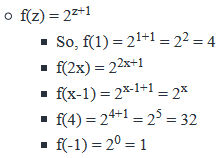• Solving the equation in terms of x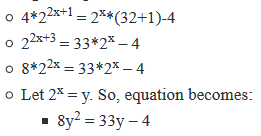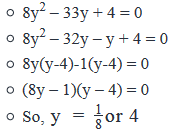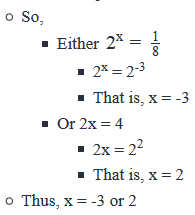Looking at the answer choices, we see that only the value in Option A corresponds to one of the roots we found. So, the correct answer is Option A

QUESTION: 4

For a positive integer x, the function f(x) is defined as f(x)=2x2+3x+4

. Is the value of the function f(x) an even number?

1. x is a multiple of 3
2. x is a prime number
Solution:

Step 1 & Step 2: Understanding the Question statement and Drawing Inferences

• The function f(x) is defined as f(x)=2x2+3x+4, where x ≥ 1
• Now in function f(x), we have three terms being added
• 1st part: 2x2
• Now any number multiplied by 2 will always result in an even number.
• So 2x2 will always be even
• 2nd part: 3x
• 3x will be even, when x is even.
• This is so because, 3 is an odd number and if x → even number, it will result in od dnumber * even number=even number
• 3x will be odd, when x is odd.
• This is so because, 3 is an odd number and if x → odd number, it will result in odd number*odd number=odd number
• 3rd part: 4
• 4 will always be even
• Now, 2x2+4=evennumber+evennumber=evennumber
• So, for f(x) to be even → 3x should be even
• 3x would be even in cases when x is even (Already established above)
• So we have to ultimately find the even-odd nature of x to find whether f(x) is even or not.

Step 3: Analyze statement 1 independently

Statement 1:

• x is a multiple of 3.
• So x can be even (Example: 6) or it can be odd (Example :3)
• So, we will not be able to determine the nature of x whether it is even or odd,
• Hence won’t be able to tell whether function f(x) will give an even value or not.
• Hence statement 1 is not sufficient to answer the question

Step 4: Analyze statement 2 independently

Statement 2:

• x is a prime number.
• So x can be an even prime number ‘2’ or it can be odd prime number like 3, 5,7.
• So, we will not be able to determine the nature of x whether it is even or odd
• Hence won’t be able to tell whether function f(x) will give an even value or not.
• Hence statement 2 is not sufficient to answer the question.

Step 5: Analyze the two statements together

• From statement 1, we have x is a multiple of 3
• From statement 2, we have x is a prime number and thus can be {2,3,5,7,….}
• Only one number 3’ will satisfy both the statements because the number ‘3’ is a multiple of 3 and is also a prime number. All other numbers except ‘3’ will be multiple of 3 and hence will not be prime.
• Now when x=3, f(x) will be odd, hence its value will not be an even number.
• So by combining both statements we are able to arrive at a unique answer.
• Hence the correct answer is option C- Both statements together are necessary to arrive at a unique answer.
QUESTION: 5

For all positive integers x,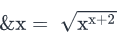when x is even and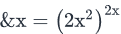when x is odd. What is the value of positive integer x?

(1) The highest power of 2 that divides &(4x) completely is 36

(2) The highest power of 2 that divides (&4)*x completely is 8

Solution:

Steps 1 & 2: Understand Question and Draw Inferences

Given:

• Integer x > 0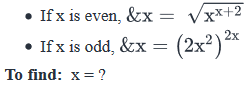Step 3: Analyze Statement 1 independently

(1) The highest power of 2 that divides &(4x) completely is 36

• This means, &(4x) contains 236 but not any higher power of 2
• 4x is definitely an even integer.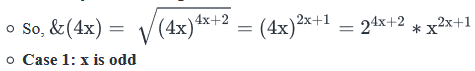• In this case, the power of 2 will only come from the term 24x+2
• Since the highest power of 2 in &(4x) is 36, we can write:
• 4x + 2 = 36
• That is, 4x = 34
• This doesn’t give an integral value of x. So, this case is rejected.
• Case 2: x is even
• In this case, x contains at least 21 but may also contain higher powers of 2.
• So, we will have to consider these possibilities: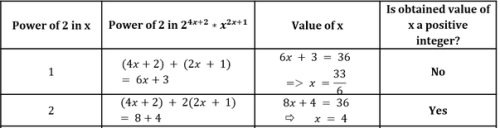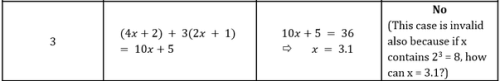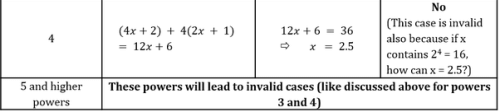• So, the only possible value of x = 22 = 4

Thus, Statement 1 is sufficient to determine a unique value of x.

Step 4: Analyze Statement 2 independently

(2) The highest power of 2 that divides (&4)*x completely is 8

• Statement 2 tells us that the highest power of 2 that divides 26x is 8
• 26 is a constant. The power of 2 in this number is 6
• So, from Statement 2, we infer that the highest power of 2 contained in x is 2
• So, x is divisible by 22 (that is, 4) but not be 23, 24 etc.
• Thus, x must be of the form 4 * odd number
• So, x = 4(2k+1) where k is a positive integer
• Values such as 4*1, 4*3, 4*5 etc. will all satisfy Statement 2

Thus, Statement 2 is not sufficient to determine a unique value of x.

Step 5: Analyze Both Statements Together (if needed)

Since we’ve already arrived at a unique answer in Step 3, this step is not required

QUESTION: 6

The function PF is defined as PF(a) = k, where k is the number of prime factors of positive integer a. If PF(x) = PF(2x)=PF(3x) = 2 and PF(y) = PF(5y) = PF(7y) = 2, where x and y are positive integers, what is the value of PF(least common multiple of x and y)?

Solution:

Given

• PF(a) = k, where k is the number of prime factors of positive integer a
• PF(x) = PF(2x)=PF(3x) = 2, where x is an integer > 0
• PF(y) = PF(5y) = PF(7y) = 2, where y is an integer > 0

To Find: PF(LCM(x, y)?

Approach

1. PF(LCM(x, y) = Number of prime factors of LCM(x, y)
1. LCM(x, y) will have the same prime factors as x and y
2. As PF(x) = 2 and PF(y) = 2, LCM(x, y) can have:
1.  a maximum of 4 prime factors if the prime factors of x and y are distinct
2. a minimum of 2 prime factors if the prime factors of x and y are same

2. For finding the prime factors of LCM(x, y), we need to know the prime factors of x and y

3. Finding prime factors of x
1. As PF(x) = PF(2x) = 2, i.e. both x and 2x have 2 prime factors
2. As PF(x) = PF(3x) = 2, i.e. both x and 3x also have 2 prime factors
3. We will use the above 2 relations to find the prime factors of x

4. Finding prime factors of y
1. As PF(y) = PF(5y) = 2, i.e. both y and 5y have 2 prime factors
2. As PF(y) = PF(7y) = 2, i.e. both y and 7y have 2 prime factors
3. We will use the above 2 relations to find the prime factors of y

5. Once we find the prime factors of x and y, we can calculate the prime factors of LCM(x, y)

Working Out

1. Finding prime factors of x
1. As PF(x) = PF(2x) = 2, i.e. both x and 2x have 2 prime factors  and 2 is one of the prime factors of x
2. As As PF(x) = PF(3x) = 2, i.e. both x and 3x also have 2 prime factors and 3 is one of the prime factors of x
3. Thus, x has 2 and 3 as its prime factors.
2. Finding prime factors of y
1. As PF(y) = PF(5y) = 2, i.e. both y and 5y have 2 prime factors and 5 is one of the prime factors of y.
2. As PF(y) = PF(7y) = 2, i.e. both y and 7y have 2 prime factors and 7 is one of the prime factors of y.
3. Thus, y has 5 and 7 as its prime factors.
3. As x and y do not have any common prime factor, LCM(x, y) will have {2, 3, 5, 7} as its prime factors.
4. Thus PF(LCM(x, y)) = 4

QUESTION: 7

For any integers x and y, min(x, y) and max(x, y) denote the minimum and the maximum of x and y, respectively. For example, min(2, 1) = 1 and max(2,1) = 2. If a, b, c and d are distinct positive integers, is max(a, max(b, min(c, d))) = max(d, max(a, min(b, c))) ?

(1) b, c and d are factors of a

(2) a – 2d = b + c

Solution:

Steps 1 & 2: Understand Question and Draw Inferences

• min(x, y) → minimum of x and y, where x and y are integers
• max(x, y) → maximum of x and y, where x and y are integers
• a, b, c, d are distinct integers > 0

To Find: Is max(a, max(b, min(c, d))) = max(d, max(a, min(b, c))) ?

Step 3: Analyze Statement 1 independently

(1) b, c and d are factors of a

• So, we can write a = bx = cy = dz, where x, y and z are distinct integers > 1
• For example, since 2, 3 and 6 are factors of 36, we can write: 36 = 2x  =3y = 6z (in this example, x, y and z will be equal to 18, 12 and 6 respectively)
• Hence, a > {b, c, d}
• Like, in the above example, 36 > {2, 3, 6}
• For solving the expression, we need to start from the innermost bracket. Since we do not know which of c or d is smaller, max(a, max(b, min(c, d))) = max(a, max(b, (c or d)))
• Now, we do not know, which of b, c or d is the greatest,  max(a, max(b, (c or d))) = max (a, (b, c or d))
• However, we do know that a > b, c, d. So, we can write  max (a, (b, c or d)) = a
• Similarly, we do not know which b or c is smaller, so, max(d, max(a, min(b, c))) = max (d, max (a, (b or c)))
• However, we do know that a > b , c. So, we can write  max (d, max (a, (b or c)))= max(d, a)
• Also, as a > d, we can write max (d, a) = a

So, max(a, max(b, min(c, d))) = max(d, max(a, min(b, c))).

Step 4: Analyze Statement 2 independently

(2) a – 2d = b + c

• Rearranging the terms: a = b + c + 2d. So, a > b, c and d
• We know that a > b, c, d and for this case we have already evaluated in statement-1 that max(a, max(b, min(c, d))) = max(d, max(a, min(b, c))).

So, max(a, max(b, min(c, d))) = max(d, max(a, min(b, c))).

Step 5: Analyze Both Statements Together (if needed)

As we have a unique answer from steps 3 and 4, this step is not required.

QUESTION: 8

The function f(x) is defined as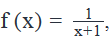where x is not equal to -1. If a is not equal to -1 or 0, which of the following expressions must be true for all values of a?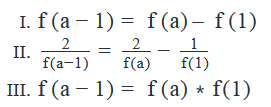Solution:

Given:

• Function f(x) is defined as• To find: Which of the 3 expressions is/are true for all values of a?

Approach:

• Since the three expressions contain f(a – 1), f(a) and f(1), we’ll first find the expressions for these 3 functions
• Then, we’ll evaluate the given expressions one by one.
• Working Out:

• Finding the expressions for f(a – 1), f(a) and f(1)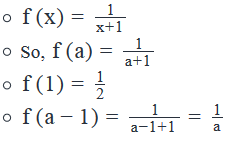• Evaluating Expression I
• f(a−1)=f(a)–f(1)
• We will assume that f(a-1) = f(a) – f(1) is true and then see if it leads to a real value of a or not.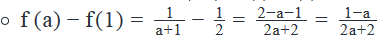• Equating the above expression with the expression for f(a-1):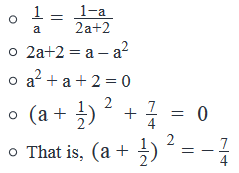• For no real value of a can the square of a+1/2  be negative
• Therefore, no real value of a satisfies the equation given in Expression I.
• So, Expression I is not true.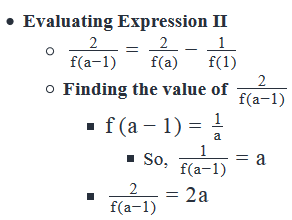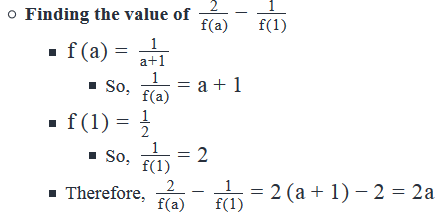• Since Left hand side of Expression II has the same value as the right hand side, this expression will hold true for all values of a.

Evaluating Expression III

• f(a−1)=f(a)∗f(1)
• The value of the left hand side of Expression III = 1/a
• The value of the right hand side of Expression III = 1/2(a+1)
•
• Equating the 2 values, we get: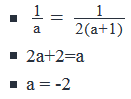• Thus, Expression III is true for only 1 value of a (-2) – not for other values.
• So, Expression III is not a must be true expression.
• Thus, we have seen that out of the 3 expressions, only Expression II is a must be true expression.

Looking at the answer choices, we see that the correct answer is Option B

QUESTION: 9

If % denotes one of the arithmetic operations, addition, subtraction, multiplication or division, and if z is an integer, what is the value of 2z%z?

(1) 3%(2z)=−(z%1)

(2) (3z)%(z−1)=5

Solution:

Steps 1 & 2: Understand Question and Draw Inferences

Given: Integer z

• % operation denotes:
• either +
• or –
• or ×
• or ÷

To find: 2z%z =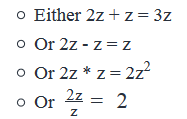So, in order to find the value of 2z%z, we need to find out what % is and (if % is not division, then) also what z is.

Step 3: Analyze Statement 1 independently

(1) 3%(2z) = - (z%1)

• Since we don’t know what operation % is, let’s consider all the 4 possibilities and see which of these satisfy the constraint that z is an integer: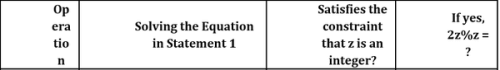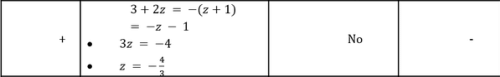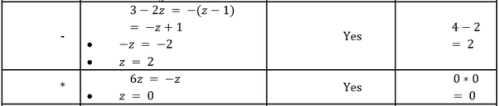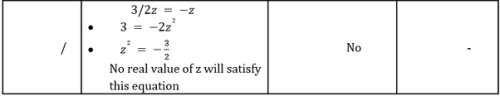As is clear from the table, we get 2 possible values of 2z%z from Statement 1.

So, Statement 1 is not sufficient.

Step 4: Analyze Statement 2 independently

(2) (3z)%(z - 1) = 5

• Since we don’t know what operation % is, let’s consider all the 4 possibilities and see which of these satisfy the constraint that z is an integer: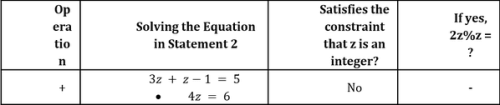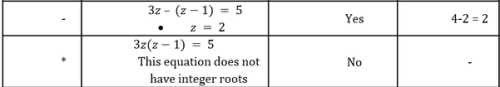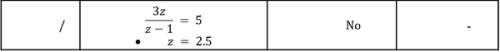This statement leads us to a unique value of the required expression.

So, this statement alone is sufficient.

Step 5: Analyze Both Statements Together (if needed)

Since we’ve already arrived at a unique answer in Step 4, this step is not required

QUESTION: 10

For all positive numbers a and b, the function {a, b} = k, where k is the multiple of b nearest to a.. If x and y are positive integers such that x/y= 15.25 and {xy,y} = 16, what is the value of y?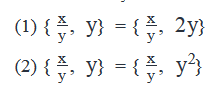Solution:

Steps 1 & 2: Understand Question and Draw Inferences

• Positive integers x and y such that {x/y,y} = 16
• As 16 is a multiple of y, possible values of y = factors of 16 = {1, 2, 4, 8, 16}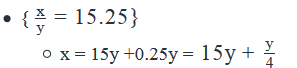• As x is an integer, y/4 has to be an integer
• Out of {1, 2, 4, 8, 16}, y/4  is an integer for y = {4, 8, 16]

To Find: Value of y

As we’ve inferred above, the possible values of y are {4, 8, 16}

Step 3: Analyze Statement 1 independently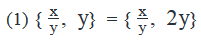Since it’s given that {xy,y} = 16, using Statement 1 we can write: {x/y,2y} = 16. So, 16 is a multiple of 2y.

• In Steps 1 and 2 above, we’ve inferred that y = {4, 8 or 16}. So, 2y = {8, 16 or 32}. But 16 is not a multiple of 32. So, 2y ≠ 32.
• Therefore, 2y = 8 or 16
• Possible values of y = {4 or 8}

Since, we do not have a unique value of y, the statement is insufficient to answer.

Step 4: Analyze Statement 2 independently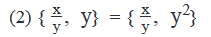Since it’s given that {xy,y} = 16, using Statement 1 we can write: {x/y,y2} = 16. So, 16 is a multiple of y2.

• In Steps 1 and 2 above, we’ve inferred that y = {4, 8 or 16}. So, y= {16, 64 or 1024}. But 16 is not a multiple of 64 or 1024. Hence y2 = 16
• Hence y = 4 (since y is given to be a positive integer, y cannot be equal to -4)

As we have a unique value of y, statement is sufficient to answer

Step 5: Analyze Both Statements Together (if needed)

As we have a unique answer from step-4, this step is not required.

QUESTION: 11

The distance travelled (D) by a car in miles when it uses more than 1 liter of fuel is given by D= x +kv, where v is the liters of fuel used, and x, and k are constants. If the car uses 4 liters of fuel to travel 53 miles and 8 liters of fuel to travel 101 miles, how much fuel in litres will the car require to travel 149 miles?

Solution:

Given

• D = x + kv, where
• D is the distance travelled and v is the liter of fuel used
• x and k are constants
• Case-I:
• 4 liters of fuel used to travel 53 miles
• Case-II:
• 8 liters of fuel used to travel 101 miles

To Find: Liters of fuel used to travel 149 miles?

• So, in the context of the equation 149 = x +kv
• We need to find the value of v

Approach

1. To find the liters of fuel used to travel 149 miles, we will need to find the values of x and k. Since there are 2 unknowns, we will need 2 equations.
2. Case-I: Using 4 liters of fuel to travel 53 miles
1. 53 = x +4k……(1)
3. Case-II: Using 8 liters of fuel to travel 101 miles
1. 101 = x + 8k….(2)
4. As we have 2 equations and 2 unknowns, we can find the value of x and k.

Working Out

(2) – (1), we have

4k = 48, i.e. k =12.

Substituting the value of k in (1), we have x = 5

Putting the values of x and k in the equation 149 = x+ kv, we have

149 = 5 +12v, i.e. v = 12 liters

Hence, it will require 12 liters to travel a distance of 149 miles.

QUESTION: 12

If [z] denotes the least integer greater than or equal to z and [z2] = 2, which of the following could be the value of [z]?

I. 2

II. 1

III. -2

Solution:

Given:

• The function [z]
• [z2] = 2

To find: Can [z] be {2, 1, -2}?

Approach:

1. To find which values of [z] are possible and which are not, we need to first know the range of possible values of z. Once we know what z can be, we’ll be able to find what [z] can be.
2. We’ll get an idea of the possible values of z from the fact that [z2] = 2

Working Out:

• [z2] = 2
• This means, the least integer that is greater than or equal to z2 is 2.
• So, the value of z2 must be equal to 2 or must lie between 1 and 2 (because if z2 is less than 1 then [z2] would be 1 or less and not 2)
• We can write: 1 < z2 ≤ 2
• The above inequality contains 2 inequalities: z2 > 1 AND z2 ≤ 2
• So now, we’ll solve these inequalities one by one, and then find the values of z that satisfy both these inequalities
• Solving z2 > 1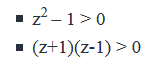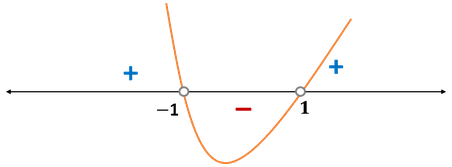• This means, z < - 1 or z > 1 . . . (1)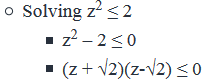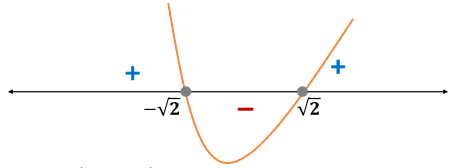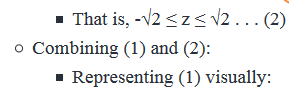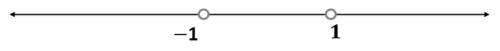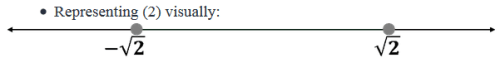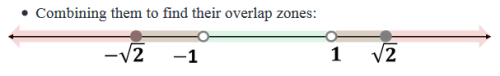• The gray zones in the above figure represent the overlap zones, that is, those values of z that satisfy both the inequalities
• So, either -√2 ≤ z < -1 or 1 < z ≤ √2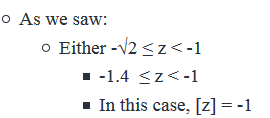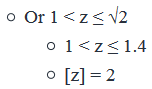Thus, we see that [z] is either equal to -1 or equal to 2. Out of the 3 given values, only value I (which is 2) is therefore possible.

Looking at the answer choices, we see that the correct answer is Option A

QUESTION: 13

For distinct positive integers x and y, where x < y, the function FP(x, y) returns the smallest prime number between x and y, exclusive, or the text string ‘NULL’ if no such number is found. If FP(a, b) +FP(c, d) = FP(e, f), where a, b, c, d, e and f are distinct positive integers, what is the value of ca ?

(1) FP(g, h) = a, where g and h are distinct positive integers

(2) c is less than the minimum possible value of the function FP(x,y).

Solution:

Steps 1 & 2: Understand Question and Draw Inferences

• For positive integers x and y where x < y, FP(x, y) returns the smallest prime number between x and y, exclusive
• FP(a, b) +FP(c, d) = FP(e, f)
• FP(a, b), FP(c, d) and FP(e, f) each return a prime number
• So, the above equation conveys that the Sum of two prime numbers is equal to another prime number
• As all prime numbers except 2 are odd, FP(e, f) will be odd and either of FP(a, b) or FP(c, d) will be even i.e. 2
• For example, if both FP(a,b) and FP(c, d) are odd, then their sum i.e. FP(e, f) will be even, i.e. 2, which is not possible as there are no prime numbers less than 2
• So, the only case possible is that either of FP(a, b) or FP(c, d) is 2 and hence the sum of FP(a, b) and FP(c, d), which is FP (e, f) is odd
• If FP(a, b) = 2
• a = 1, ca = c
• If FP(c, d) = 2
• If c = 1, ca = 1

To Find: Value of c

Step 3: Analyze Statement 1 independently

(1) FP(g, h) = a, where g and h are distinct positive integers

• Since we know that FP(g, h) will return a prime number, we can infer that a is a prime number
• As a is a prime number, a > 1. So, FP(a, b) ≠ 2
• Hence FP(c, d) = 2
• For this to be possible, c = 1

If c = 1, ca = 1

Step 4: Analyze Statement 2 independently

(2) c is less than the minimum possible value of the function FP(x,y).

• Minimum possible value of the function FP(x, y) = smallest prime number = 2
• So, c < 2,
• As c is given to be a positive integer and now we know that c < 2, the only possible value of c = 1

Hence ca = 1

Step 5: Analyze Both Statements Together (if needed)

As we have a unique answer from steps 3 and 4, this step is not required.

QUESTION: 14

If the operation a* is defined by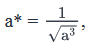what is the value of

Solution:

Given:

• Operation a* is defined by• To find: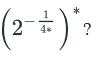Approach:

1. We’ll apply the definition of operation a* on the given expression to calculate the value that the expression results in.
• Note that the given expression applies the operation a* twice: first in the power of 2 within the brackets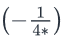and then to the whole expression inside the brackets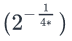• So, we will first find the value ofand use it to find the value of• Next, we will find the value ofWorking Out: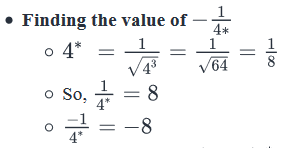• Finding the value of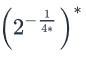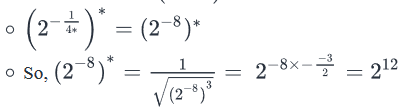• Looking at the answer choices, we see that the correct answer is Option E
QUESTION: 15

For any non-zero numbers p and q,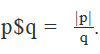What is the value of p\$q?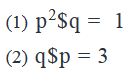Solution:

Steps 1 & 2: Understand Question and Draw Inferences

Given: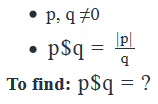In order to find the required value, we need to know the value of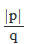Step 3: Analyze Statement 1 independently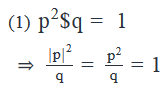=> q= p2  ( Since |p|2 is always positive, we can remove the modulus sign)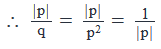Since we do not know the value of |p|, we cannot find a unique value ofStatement 1 is not sufficient.

Step 4: Analyze Statement 2 independently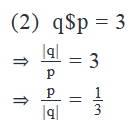• However, q may be positive or negative
• This equation conveys that p is positive. So, |p| = p
•=13(if q is positive) or − 13 (if q is negative)

Thus, Statement 2 is not sufficient to find a unique value of the ratioStep 5: Analyze Both Statements Together (if needed)

• From Statement 1: q = p2

• From Statement 2:=13 (if q is positive ) or − 13 ( if q is negative)
• Since q is the square of a non-zero number, q must be positive
• Combining both statements:=13 (since q is positive )

Thus, the two statements together are sufficient to obtain a unique value ofQUESTION: 16

A function f(x) is defined as f(x) = x + x2  for an integer x. If a is an integer, is f(a) a prime number?

1. f(a)+f(a+1) is a prime number

2. The greatest common divisor of f(3) & f(4) is f(a)*2.

Solution:

Step 1 & Step 2: Understanding the Question statement and Drawing Inferences

Given Info:

• A function f(x) is defined as f(x) = x + x2 , where x is an integer.
• So function f(x) is given as a quadratic function in x and can be written as
f(x) = x(x+1)

To find:

• Is f(a) a prime number?
• So f(a) = a(a+1)
• Now f(a) is the product of two consecutive numbers.
• Product of any two consecutive number will always have one even number and one odd number and thus the product will always be an even number. →
EvenNumber*OddNumber=EvenNumber
• , and the only even number which is a prime number is 2.
• So, f(a) will be prime only when f(a) = 2
• So we have to find the values of a, for which f(a)=2, to determine whether f(a) is a prime number or not.
• If f(a) = 2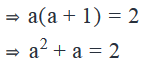Subtracting 2 from both sides of the equation, we get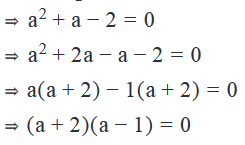• So this gives either a=-2 or a=1
• So for a = -2 or a=1 the above expression of f(a) will be a prime number otherwise it will be not. So from the given statements we will determine the value of a and then conclude whether f(a) is a prime number or not.

Step 3: Analyze statement 1 independently

Statement 1:

• f(a)+f(a+1) is a prime number
• We have, f(a)=a(a+1) (Already established above)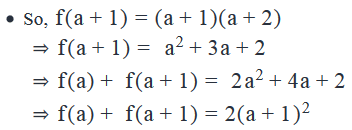• The above expression will be prime when (a+1)2=1 or f(a) + f(a+1) = 2 . This is so because multiplication of (a+1)2 with 2 will always result in even number and the only even number which is prime is 2.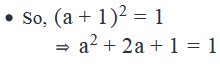• Subtracting 1 from both sides of the equation, we get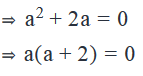• This means either a=0 or a=-2
• Now f(a) will be prime in case when a=-2 but it will not be prime when a=0 (As already established above)
• Hence statement 1 is not sufficient to answer the question

Step 4: Analyze statement 2 independently

Statement 2:

• The greatest common divisor of f(3) & f(4) is f(a)*2.
• Now, we know  f(x)=x(x+1)
• So,f(3)=3(3+1)=12
• And,f(4)=4(4+1)=20
• Now 12 can be expressed in prime factors as → 12 = 3*22
• And 20 can be expresses in prime factors as → 20 = 5*22
• The greatest common factor of 12 and 20 is 22.
• So the greatest common divisor of f(3) and f(4) will be equal to 4.
• Now we are given this greatest common divisor is equal to f(a) * 2
• So we have, f(a) * 2 = 4

⇒ f(a) = 2

• Now since f(a) = 2, we will have f(a) is a prime number, because 2 is a prime number.
• Hence statement 2 is sufficient to arrive at a unique answer.

Step 5: Analyze the two statements together

• Since from statement 2 we are able to arrive at a unique answer (as shown in step 4) combining and analysing both statements together is not required.

Hence the correct answer is option B- Only statement 2 is sufficient to arrive at a unique answer.

QUESTION: 17

The function Ro rounds a positive decimal number to its nearest integer starting from its rightmost digit. What is the value of Ro(64.4ab5), where a and b are single digit positive integers?

(1) Ro(36.4a7) = 37

(2) Ro(87.b6) = 88

Solution:

Steps 1 & 2: Understand Question and Draw Inferences

• Function Ro rounds a positive decimal number to its nearest integer starting from its rightmost digit
• a and b are single digit positive integers
• 0 < a, b < 10

To Find: value of Ro(64.4ab5)

• As the function Ro rounds off the number starting from its rightmost digit, in the first step Ro(64.4ab5) = Ro(64.4a(b+1)). This is because the last digit is 5 and hence 64. 4ab5 will be rounded up to 64.4a(b+1).
• Now, the final value of Ro(64.4a(b+1)) = 64 or 65. Let’s evaluate the possible cases:
• Ro(64.4a(b+1)) = 64. This will be possible when Ro(64.4a) = Ro(64.4). So, for all the cases where a < 4, Ro(64. 4a) = Ro(64.4) = 64. Please note that if a < 4, the value of b is immaterial, because even if b ≥ 5, the maximum value of a = 4, in which case Ro(64.4a) = Ro(64.4) = 64
• Ro64.4a(b+1)) = 65. This will be possible if Ro(64.4a) = Ro(64.5). This can happen in following ways:
• If a ≥ 5 and b is any value OR
• a =4 and b=>4, then Ro(64.4a(b+1)) = Ro(64.4(a+1)) = Ro(64.45) = Ro(64.5) = 65

Step 3: Analyze Statement 1 independently

(1)Ro(36.4a7) = 37

• Since the function Ro rounds off starting from the rightmost digit, the first digit that will be rounded off will be a. The rounding of a will depend on the value to the right of it, i.e. 7. So, we have Ro(36.4a7) = Ro(36.4(a+1))
• For Ro(36.4(a+1)) = 37, Ro(36.4(a+1)) = Ro(36.5), i.e. 4 should be rounded up to 5. This will be possible when a+1 ≥ 5, i.e. a ≥ 4

If a = 4, we need to know the value of b to find the value of Ro(64.4a(b+1))

If a > 4, Ro(64.4a(b+1)) = 65

As we do not have a unique answer, the statement is insufficient to answer.

Step 4: Analyze Statement 2 independently

(2) Ro(87.b6) = 88

• Since the function Ro rounds off starting from the rightmost digit, the first digit that will be rounded off will be b. The rounding of b will depend on the value to the right of it, i.e. 6. So, we have Ro(87.b6) = Ro(87.(b+1)) = 88
• So, b+1 ≥ 5, i.e. b ≥ 4
• Using the value of b in Ro(64.4a(b+1)) = Ro(64. 4(a+1))

Need to know the value of a. Insufficient to answer

Step 5: Analyze Both Statements Together (if needed)

(1) From Statement 1, a ≥ 4

(2) From Statement 2, b ≥ 4

• Ro(64.4ab5) = Ro(64.4a(b+1))
• As b ≥ 4, b +1 ≥5. So,  Ro(64.4a(b+1)) = Ro(64.4(a+1))
• Since a ≥ 4, a +1 ≥5. So, Ro(64.4(a+1)) = Ro(64.5) = 65

QUESTION: 18

The operation @ is defined for all distinct and nonzero a and b by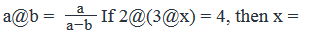Solution:

Given: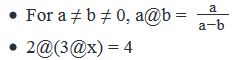To find: x = ?

Approach:

1. We’ll first evaluate the expression for 3@x
2. Then, we’ll evaluate the expression for 2@(3@x), and finally, will equate the resulting expression with 4. We’ll thus get an equation in x, upon solving which, we’ll get the value of x.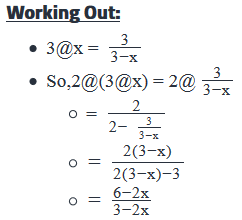• Since we’re given that 2@(3@x) = 4, we can write: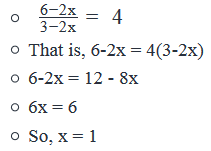Looking at the answer choices, we see that the correct answer is Option D

QUESTION: 19

The symbols @ and # each denote one of the four arithmetic operations: addition, subtraction, multiplication and division. If the symbols denote different arithmetic operations such thatand a@b#c = c#b@a, where a, b and c are distinct positive integers, what is the value of 4@5#4?

Solution:

Given

• @ = { + , - , *, /}
• # = { + , - , *, /}
• @ ≠ #
• a@b#c = c#b@a, where a, b , c are distinct integers > 0

To Find: value of 4@5#4?

• We need to find the operations that @ and # denote.

Approach

1. To find the operations that @ and # denote, we will analyse the relation a@b#c = c#b@a.
2. We will calculate the values of 4@5#4 for all the possible operations denoted by @ and #.

Working Out

1. As a@(b#c) = (c#b)@a, we can say that @ = {*, +}
2. Similarly, as (a@b) # c = c#(b@a), we can say that # = {+, *}
3. Now, let’s evaluate all the possible cases for the operations denoted by @ and #.
• {@, #} = {*, +} or {*, *} or {+, *} or {+, +}.
• Since @ and # denote different operations, {*,*} and {+, +} are not the possible cases.
4. Evaluating the possible cases, we have:
1. If @ = * and # = +
1. So, 4@5#4 = 4*5 + 4 = 24
2. If @ = + and # = *
1. So, 4@5#4 = 4+5*4 = 24

Thus, we have a unique value of 4@5#4 = 24

QUESTION: 20

A function f(x) is defined as f(x)=3x2−20x+c, where c is a constant. Also given f(1) =  -16. What is the value of f(c) + f(-c) ?

Solution:

Given Info:

• Function f(x) is given as f(x)=3x2−20x+c
• , where c is a constant
• The above function is a quadratic function in x
• Also given f(1)=−16

To Find:

• Value of f(c)+f(−c)

⇒f(c)+f(−c)=6c2+2c

Approach:

Working out:

• Now, f(c)=3c2−20c+c
• (Putting value of c in the given function → f(x)=3x2−20x+c
• And, f(−c)=3c2+20c+c
• Adding both functions, we get
⇒f(c)+f(−c)=3c2−20c+c+3c2+20c+c
• Now in order to calculate the above value, we need to determine the value of c.
• To determine the value of c, we will work on the quadratic function in x. We know the value of function at x=1 as’ -16’ as given in the question, so we will calculate the value of c by putting the value of f(x) and the value of x.
• After knowing the value of c from the given value of the function at x=1, we will find the value of f(c)+f(−c)
• f(x)=3x2−20x+c
• Given f(1)=−16

⇒ f(1)=3(12)−20(1)+c

⇒ f(1)=c−17

• Now f(1)=−16

⇒ c−17=−16

⇒ c=1

• Now we have already established above, f(c)+f(−c)=6c2+2c
• Putting value of c from above, we get

⇒ f(c)+f(−c)=6(1)2+2(1)

⇒ f(c)+f(−c)=8Use Code STAYHOME200 and get INR 200 additional OFF Use Coupon Code